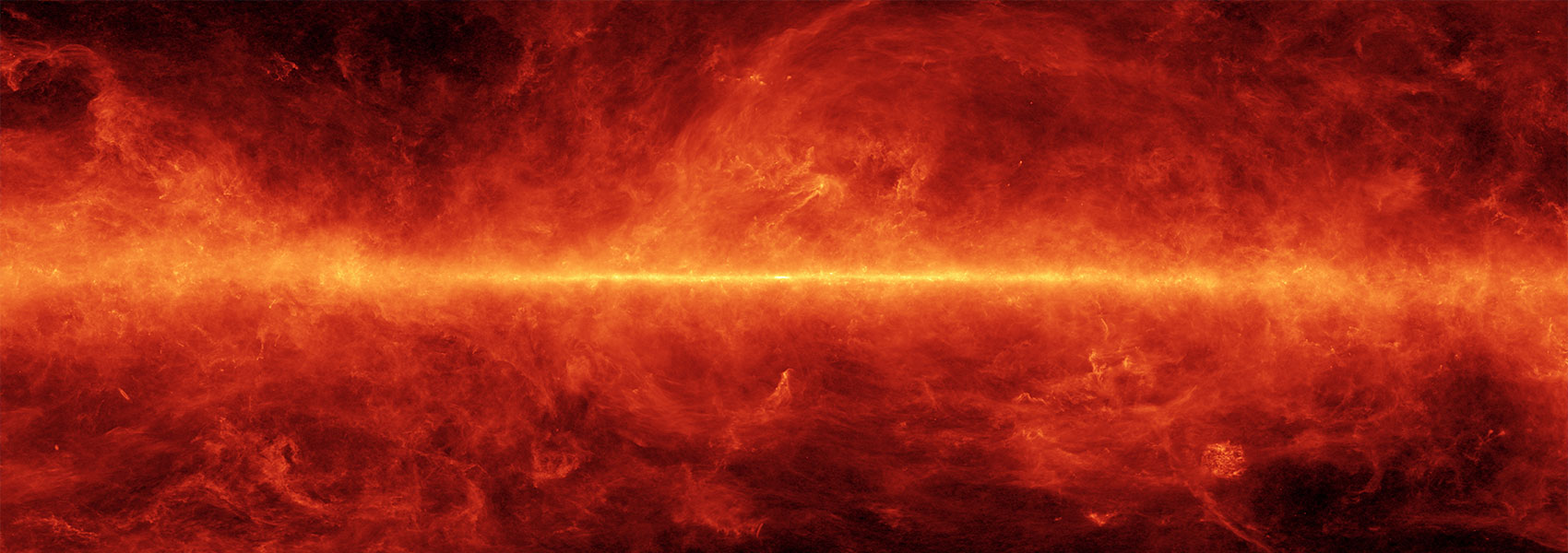# The Direct-method Oxygen Abundance of Typical Dwarf Galaxies at Cosmic High Noon

May 2023 • 2023ApJ...948..108G

Authors • Gburek, Timothy • Siana, Brian • Alavi, Anahita • Emami, Najmeh • Richard, Johan • Freeman, William R. • Stark, Daniel P. • Snapp-Kolas, Christopher

Abstract • We present a Keck/MOSFIRE rest-optical composite spectrum of 16 typical gravitationally lensed star-forming dwarf galaxies at 1.7 ≲ z ≲ 2.6 (z mean = 2.30), all chosen independent of emission-line strength. These galaxies have a median stellar mass of $\mathrm{log}{({M}_{* }/{M}_{\odot })}_{\mathrm{med}}={8.29}_{-0.43}^{+0.51}$ and a median star formation rate of ${{\rm{S}}{\rm{F}}{\rm{R}}}_{{\rm{H}}\alpha }^{{\rm{m}}{\rm{e}}{\rm{d}}}={2.25}_{-1.26}^{+2.15}\,{M}_{\odot }\,{{\rm{y}}{\rm{r}}}^{-1}$ . We measure the faint electron-temperature-sensitive [O III] λ4363 emission line at 2.5σ (4.1σ) significance when considering a bootstrapped (statistical-only) uncertainty spectrum. This yields a direct-method oxygen abundance of $12+\mathrm{log}{({\rm{O}}/{\rm{H}})}_{\mathrm{direct}}={7.88}_{-0.22}^{+0.25}$ ( ${0.15}_{-0.06}^{+0.12}\ {Z}_{\odot }$ ). We investigate the applicability at high z of locally calibrated oxygen-based strong-line metallicity relations, finding that the local reference calibrations of Bian et al. best reproduce (≲0.12 dex) our composite metallicity at fixed strong-line ratio. At fixed M *, our composite is well represented by the z ~ 2.3 direct-method stellar mass-gas-phase metallicity relation (MZR) of Sanders et al. When comparing to predicted MZRs from the IllustrisTNG and FIRE simulations, having recalculated our stellar masses with more realistic nonparametric star formation histories $(\mathrm{log}{({M}_{* }/{M}_{\odot })}_{\mathrm{med}}={8.92}_{-0.22}^{+0.31})$ , we find excellent agreement with the FIRE MZR. Our composite is consistent with no metallicity evolution, at fixed M * and SFR, of the locally defined fundamental metallicity relation. We measure the doublet ratio [O II] λ3729/[O II] λ3726 = 1.56 ± 0.32 (1.51 ± 0.12) and a corresponding electron density of ${n}_{e}={1}_{-0}^{+215}\ {\mathrm{cm}}^{-3}$ ( ${n}_{e}={1}_{-0}^{+74}\ {\mathrm{cm}}^{-3}$ ) when considering the bootstrapped (statistical-only) error spectrum. This result suggests that lower-mass galaxies have lower densities than higher-mass galaxies at z ~ 2. The data presented herein were obtained at the W. M. Keck Observatory, which is operated as a scientific partnership among the California Institute of Technology, the University of California, and the National Aeronautics and Space Administration. The Observatory was made possible by the generous financial support of the W. M. Keck Foundation. Based on observations made with the NASA/ESA Hubble Space Telescope, obtained from the Data Archive at the Space Telescope Science Institute, which is operated by the Association of Universities for Research in Astronomy, Inc., under NASA contract NAS5-26555. These observations are associated with programs #9289, #11710, #11802, #12201, #12931, #13389, #13498, #13504.